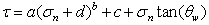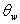Join one of our upcoming courses taking place around the world! Explore now

# Power Curve

The Power Curve model for shear-strength can be expressed as:where:

• a, b and c are parameters typically obtained from a least-squares regression fit of data obtained from small-scale shear tests. The d parameter represents the tensile strength. If included, it must be entered as a positive value.
• If you are using the Power Curve to model the shear strength of a joint, then the parameteris the "waviness angle", which is defined as follows.

## Waviness Angle

Waviness is a parameter that can be included in calculations of the shear strength of a joint or failure plane. It accounts for the waviness (undulations) of the joint surface, observed over distances on the order of 1 m to 10 m. [Miller (1988)] The waviness angle is equal to the AVERAGE dip of a failure plane, minus the MINIMUM dip of the failure plane. A non-zero waviness angle, will always increase the effective shear strength of the failure plane.

If you are NOT modelling the strength of a joint, then you can simply set the waviness angle = 0, and this term in the Power Curve equation will NOT contribute to the shear strength.

## Tensile Strength

See the Tensile Strength topic.